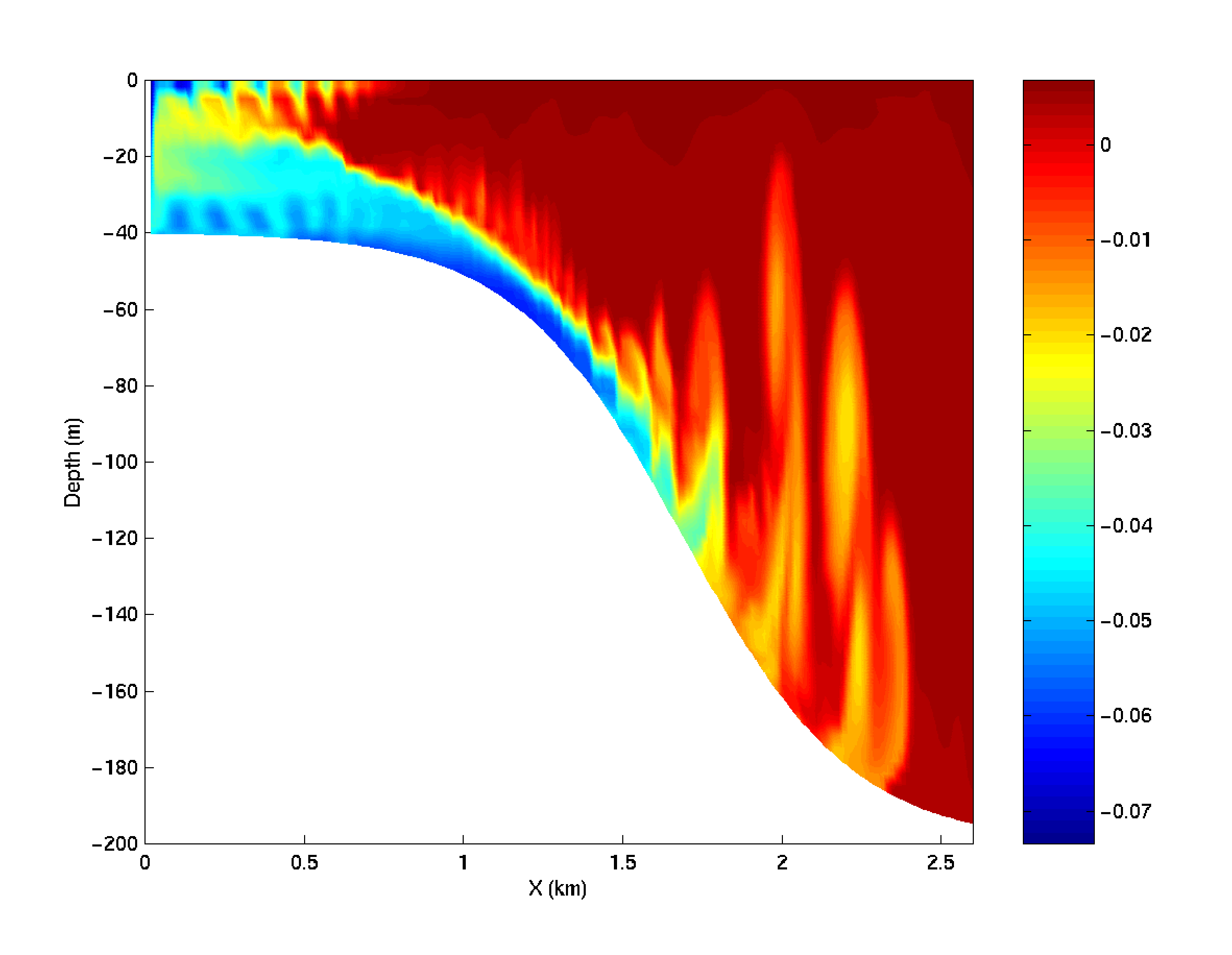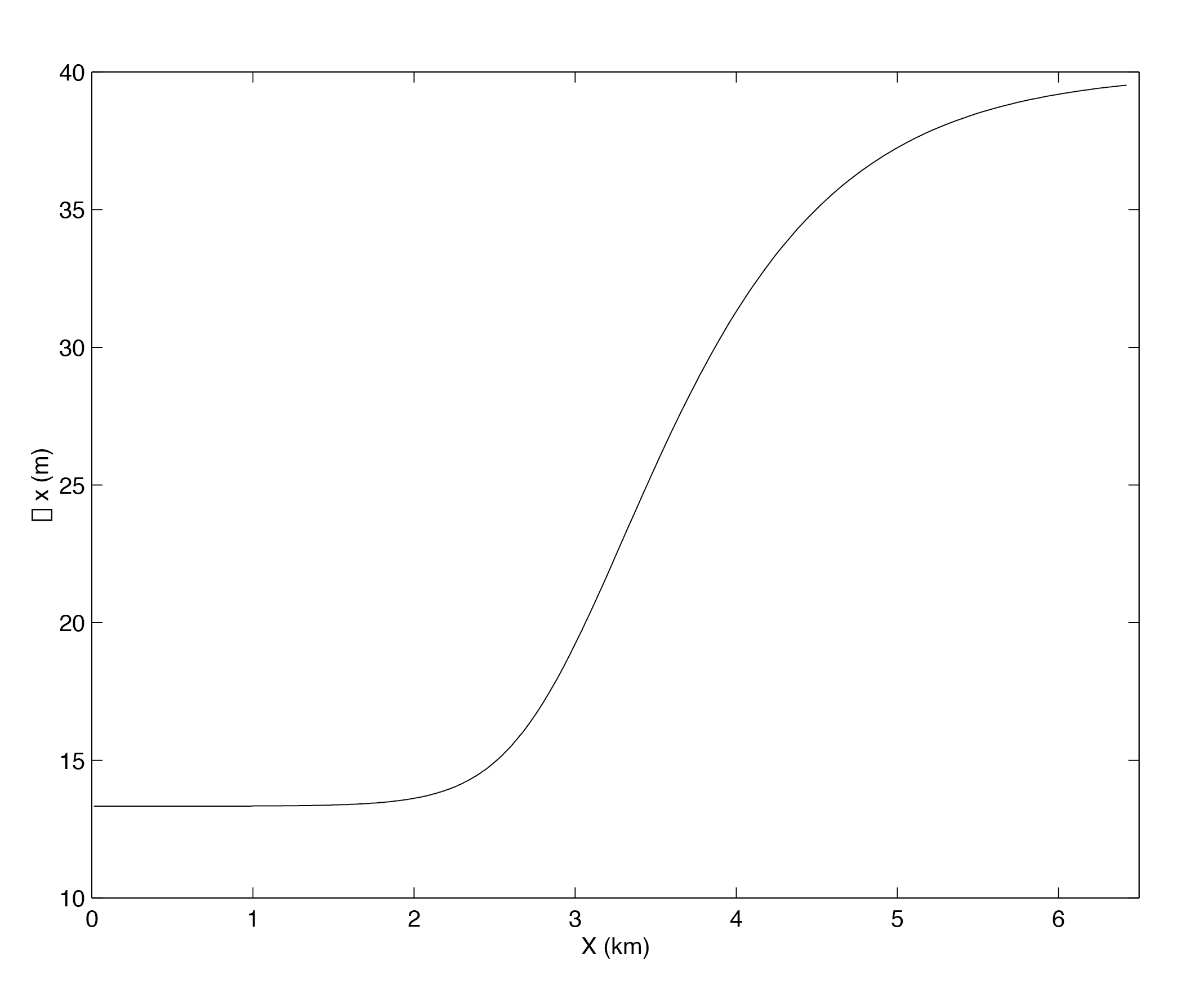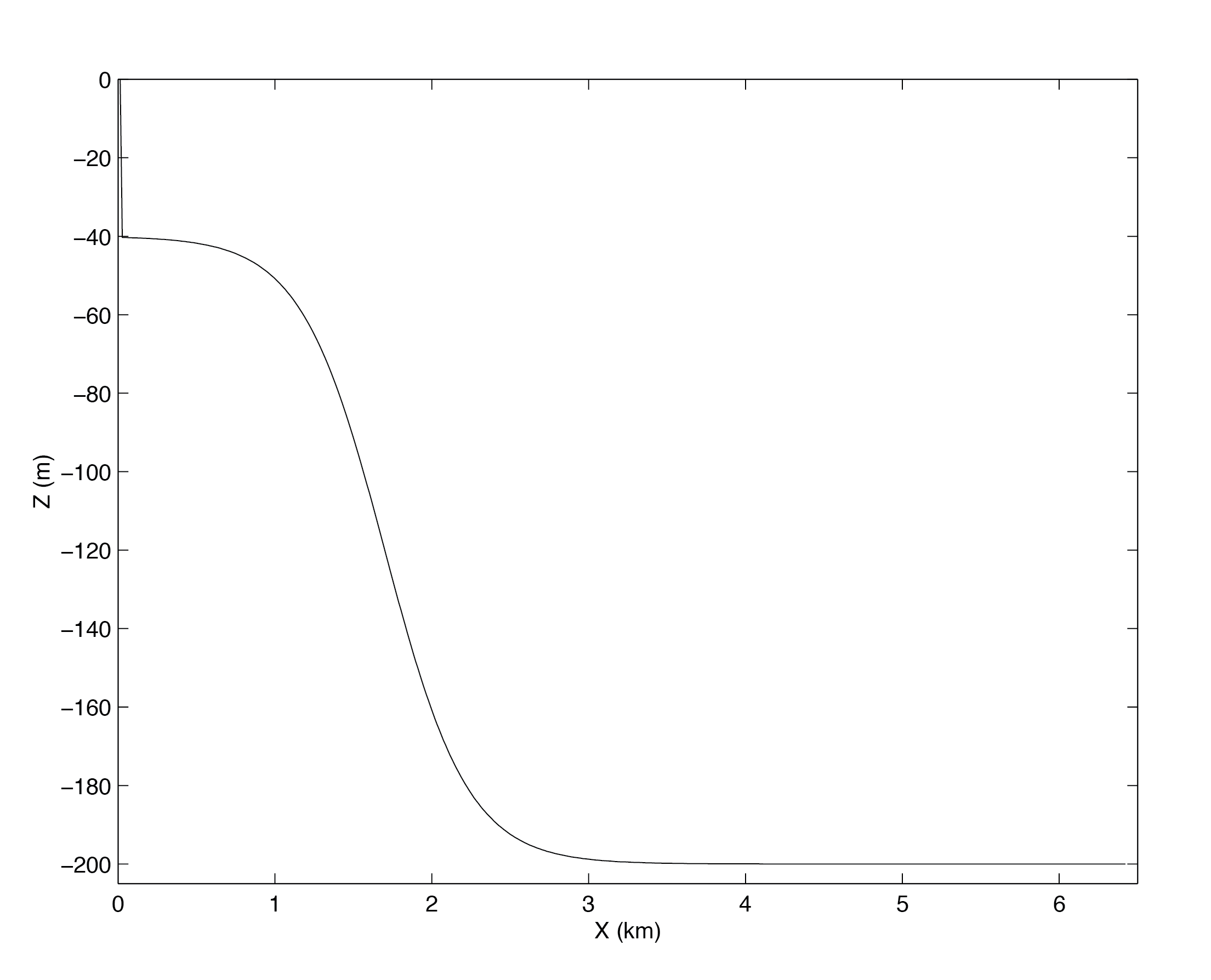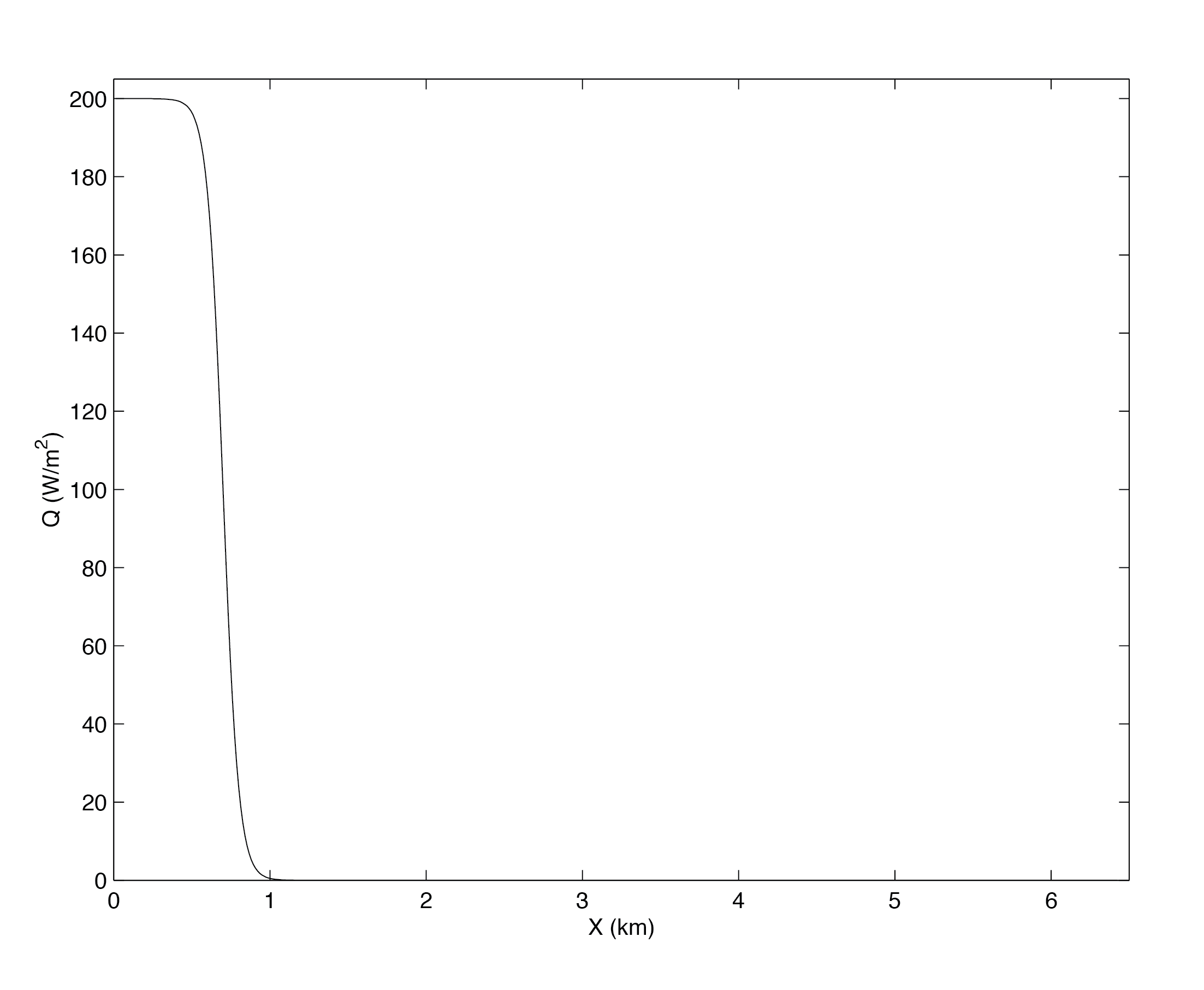# 4.9. Gravity Plume On a Continental Slope¶

(in directory: verification/tutorial_plume_on_slope/)Figure 4.42 Temperature after 23 hours of cooling. The cold dense water is mixed with ambient water as it accelerates down the slope and hence is warmer than the unmixed plume.¶

An important test of any ocean model is the ability to represent the flow of dense fluid down a slope. One example of such a flow is a non-rotating gravity plume on a continental slope, forced by a limited area of surface cooling above a continental shelf. Because the flow is non-rotating, a two dimensional model can be used in the across slope direction. The experiment is non-hydrostatic and uses open-boundaries to radiate transients at the deep water end. (Dense flow down a slope can also be forced by a dense inflow prescribed on the continental shelf; this configuration is being implemented by the DOME (Dynamics of Overflow Mixing and Entrainment) collaboration to compare solutions in different models).

The fluid is initially unstratified. The surface buoyancy loss $$B_0$$ (dimensions of L$$^2$$T$$^{-3}$$) over a cross-shelf distance $$R$$ causes vertical convective mixing and modifies the density of the fluid by an amount

$\Delta \rho = \frac{B_0 \rho_0 t}{g H}$

where $$H$$ is the depth of the shelf, $$g$$ is the acceleration due to gravity, $$t$$ is time since onset of cooling and $$\rho_0$$ is the reference density. Dense fluid slumps under gravity, with a flow speed close to the gravity wave speed:

$U \sim \sqrt{g' H} \sim \sqrt{ \frac{g \Delta \rho H}{\rho_0} } \sim \sqrt{B_0 t}$

A steady state is rapidly established in which the buoyancy flux out of the cooling region is balanced by the surface buoyancy loss. Then

$U \sim (B_0 R)^{1/3} \mbox{ ; } \Delta \rho \sim \frac{\rho_0}{g H} (B_0 R)^{2/3}$

The Froude number of the flow on the shelf is close to unity (but in practice slightly less than unity, giving subcritical flow). When the flow reaches the slope, it accelerates, so that it may become supercritical (provided the slope angle $$\alpha$$ is steep enough). In this case, a hydraulic control is established at the shelf break. On the slope, where the Froude number is greater than one, and gradient Richardson number (defined as $$Ri \sim g' h^*/U^2$$ where $$h^*$$ is the thickness of the interface between dense and ambient fluid) is reduced below 1/4, Kelvin-Helmholtz instability is possible, and leads to entrainment of ambient fluid into the plume, modifying the density, and hence the acceleration down the slope. Kelvin-Helmholtz instability is suppressed at low Reynolds and Peclet numbers given by

$Re \sim \frac{U h}{ \nu} \sim \frac{(B_0 R)^{1/3} h}{\nu} \mbox{ ; } Pe = Re Pr$

where $$h$$ is the depth of the dense fluid on the slope. Hence this experiment is carried out in the high Re, Pe regime. A further constraint is that the convective heat flux must be much greater than the diffusive heat flux (Nusselt number $$\gg 1$$). Then

$Nu = \frac{U h^* }{\kappa} \gg 1$

Finally, since we have assumed that the convective mixing on the shelf occurs in a much shorter time than the horizontal equilibration, this implies $$H/R \ll 1$$.

Hence to summarize the important non-dimensional parameters, and the limits we are considering:

$\frac{H}{R} \ll 1 \mbox{ ; } Re \gg 1 \mbox{ ; } Pe \gg 1 \mbox{ ; } Nu \gg 1 \mbox{ ; } \mbox{ ; } Ri < 1/4 .$

In addition we are assuming that the slope is steep enough to provide sufficient acceleration to the gravity plume, but nonetheless much less that 1:1, since many Kelvin-Helmholtz billows appear on the slope, implying horizontal length scale of the slope $$\gg$$ the depth of the dense fluid.

## 4.9.1. Configuration¶

The topography, spatial grid, forcing and initial conditions are all specified in binary data files generated using matlab script verification/tutorial_plume_on_slope/input/gendata.m and detailed in Section 4.9.2. Other model parameters are specified in input/data and input/data.obcs and detailed in Section 4.9.4.

## 4.9.2. Binary input data¶Figure 4.43 Horizontal grid spacing, $$\Delta x$$, in the across-slope direction for the gravity plume experiment.¶Figure 4.44 Topography, $$h(x)$$, used for the gravity plume experiment.¶Figure 4.45 Upward surface heat flux, $$Q(x)$$, used as forcing in the gravity plume experiment.¶

The domain is $$200$$ m deep and $$6.4$$ km across. Uniform resolution of $$60\times3^1/_3$$ m is used in the vertical and variable resolution of the form shown in Figure 4.43 with 320 points is used in the horizontal. The formula for $$\Delta x$$ is:

$\Delta x(i) = \Delta x_1 + ( \Delta x_2 - \Delta x_1 ) ( 1 + \tanh{\left(\frac{i-i_s}{w}\right)} ) /2$

where

\begin{split}\begin{aligned} Nx & = 320 \\ Lx & = 6400 \;\; \mbox{(m)} \\ \Delta x_1 & = \frac{2}{3} \frac{Lx}{Nx} \;\; \mbox{(m)} \\ \Delta x_2 & = \frac{Lx/2}{Nx-Lx/(2 \Delta x_1)} \;\; \mbox{(m)} \\ i_s & = Lx/( 2 \Delta x_1 ) \\ w & = 40\end{aligned}\end{split}

Here, $$\Delta x_1$$ is the resolution on the shelf, $$\Delta x_2$$ is the resolution in deep water and $$Nx$$ is the number of points in the horizontal.

The topography, shown in Figure 4.44, is given by:

$H(x) = -H_o + (H_o - h_s) ( 1 + \tanh{\left(\frac{x-x_s}{L_s}\right)} ) / 2$

where

\begin{split}\begin{aligned} H_o & = 200 \;\; \mbox{(m)} \\ h_s & = 40 \;\; \mbox{(m)} \\ x_s & = 1500 + Lx/2 \;\; \mbox{(m)} \\ L_s & = \frac{(H_o - h_s)}{2 s} \;\; \mbox{(m)} \\ s & = 0.15\end{aligned}\end{split}

Here, $$s$$ is the maximum slope, $$H_o$$ is the maximum depth, $$h_s$$ is the shelf depth, $$x_s$$ is the lateral position of the shelf-break and $$L_s$$ is the length-scale of the slope.

The forcing is through heat loss over the shelf, shown in Figure 4.45 and takes the form of a fixed flux with profile:

$Q(x) = Q_o ( 1 + \tanh{\left(\frac{x - x_q}{L_q}\right)} ) / 2$

where

\begin{split}\begin{aligned} Q_o & = 200 \;\; \mbox{(W m^{-2})} \\ x_q & = 2500 + Lx/2 \;\; \mbox{(m)} \\ L_q & = 100 \;\; \mbox{(m)}\end{aligned}\end{split}

Here, $$Q_o$$ is the maximum heat flux, $$x_q$$ is the position of the cut-off, and $$L_q$$ is the width of the cut-off.

The initial temperature field is unstratified but with random perturbations, to induce convection early on in the run. The random perturbation are calculated in computational space and because of the variable resolution introduce some spatial correlations, but this does not matter for this experiment. The perturbations have range $$0-0.01$$ $$^{\circ}\mathrm{K}$$.

## 4.9.3. Code configuration¶

The computational domain (number of gridpoints) is specified in code/SIZE.h and is configured as a single tile of dimensions $$320\times1\times60$$.

To compile the model code for this experiment, the non-hydrostatic algorithm needs to be enabled, and the open-boundaries package (pkg/obcs) is required:

## 4.9.4. Model parameters¶

Table 4.1 Model parameters used in the gravity plume experiment.

Parameter

Value

Description

$$g$$

9.81 m s-2

acceleration due to gravity

$$\rho_o$$

999.8 kg m-3

reference density

$$\alpha$$

2 $$\times$$ 10-4 K-1

expansion coefficient

$$A_h$$

1 $$\times$$ 10-2 m2 s-1

horizontal viscosity

$$A_v$$

1 $$\times$$ 10-3 m2 s-1

vertical viscosity

$$\kappa_h$$

0 m2 s-1

(explicit) horizontal diffusion

$$\kappa_v$$

0 m2 s-1

(explicit) vertical diffusion

$$\Delta t$$

20 s

time step

$$\Delta z$$

3.33333 m

vertical grid spacing

$$\Delta x$$

13.3333 - 39.5 m

horizontal grid spacing

The model parameters (Table 4.1) are specified in input/data and if not assume the default values as defined in Section 3.8. A linear equation of state is used, eosType =’LINEAR’, but only temperature is active, sBeta =0.E-11. For the given heat flux, $$Q_o$$, the buoyancy forcing is $$B_o = \frac{g \alpha Q}{\rho_o c_p} \sim 10^{-7}$$ m2 s-3. Using $$R=10^3$$ m, the shelf width, this gives a velocity scale of $$U\sim 5 \times 10^{-2}$$ m s-1 for the initial front but will accelerate by an order of magnitude over the slope. The temperature anomaly will be of order $$\Delta \theta \sim 3 \times 10^{-2}$$ K. The viscosity is constant and gives a Reynolds number of $$100$$, using $$h=20$$ m for the initial front and will be an order magnitude bigger over the slope. There is no explicit diffusion but a non-linear advection scheme is used for temperature which adds enough diffusion so as to keep the model stable. The time-step is set to $$20$$ s and gives Courant number order one when the flow reaches the bottom of the slope.